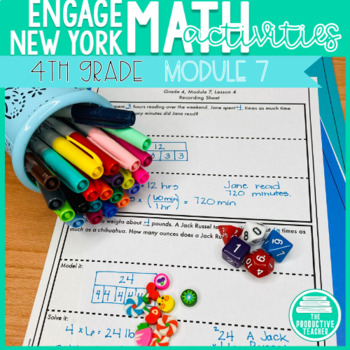# 4th Grade Math Engage New York Aligned Activities: Module 7Subject
Resource Type
File Type

PDF

(3 MB|29 pages)
Product Rating
Standards
Also included in:
1. Are you looking for a way to bring more engagement to Engage New York or Eureka Math? These activity pages give students more practice with all of the topics covered in Engage New York Grade 4.You get one activity per lesson in the module. Each activity is interactive. Students sort and match cards,
\$48.00
\$38.00
Save \$10.00
• Product Description
• StandardsNEW

Are you looking for a way to bring more engagement to Engage New York or Eureka Math? These activity pages give students more practice with all of the topics covered in Engage New York Grade 4, Module 7.

You get one activity per lesson in the module. Each activity is interactive. Students sort and match cards, generate numbers using dice, and solve unique problems to practice the learning target of the lesson. They can show their work in their math notebooks or on the provided recording sheets.

Students can repeat the activity as many times as necessary because they are creating the problems as they work. This program combines the ease of worksheets with the fun of interactive activities.

Covered in this module:

⭐ measurement

⭐ conversions

Do you want another module?

Remember to follow my store to be the first to know about all my new products! I list all my new products at 50% off for the first 24 hours!

Solve multistep word problems posed with whole numbers and having whole-number answers using the four operations, including problems in which remainders must be interpreted. Represent these problems using equations with a letter standing for the unknown quantity. Assess the reasonableness of answers using mental computation and estimation strategies including rounding.
Multiply or divide to solve word problems involving multiplicative comparison, e.g., by using drawings and equations with a symbol for the unknown number to represent the problem, distinguishing multiplicative comparison from additive comparison.
Interpret a multiplication equation as a comparison, e.g., interpret 35 = 5 × 7 as a statement that 35 is 5 times as many as 7 and 7 times as many as 5. Represent verbal statements of multiplicative comparisons as multiplication equations.
Use the four operations to solve word problems involving distances, intervals of time, liquid volumes, masses of objects, and money, including problems involving simple fractions or decimals, and problems that require expressing measurements given in a larger unit in terms of a smaller unit. Represent measurement quantities using diagrams such as number line diagrams that feature a measurement scale.
Know relative sizes of measurement units within one system of units including km, m, cm; kg, g; lb, oz.; l, ml; hr, min, sec. Within a single system of measurement, express measurements in a larger unit in terms of a smaller unit. Record measurement equivalents in a two-column table. For example, know that 1 ft is 12 times as long as 1 in. Express the length of a 4 ft snake as 48 in. Generate a conversion table for feet and inches listing the number pairs (1, 12), (2, 24), (3, 36),...
Total Pages
29 pages
Not Included
Teaching Duration
1 month
Report this Resource to TpT
Reported resources will be reviewed by our team. Report this resource to let us know if this resource violates TpT’s content guidelines.